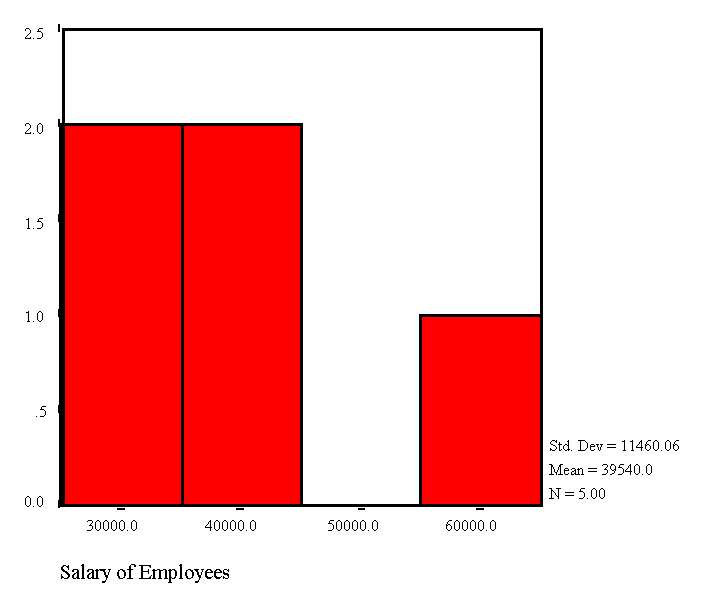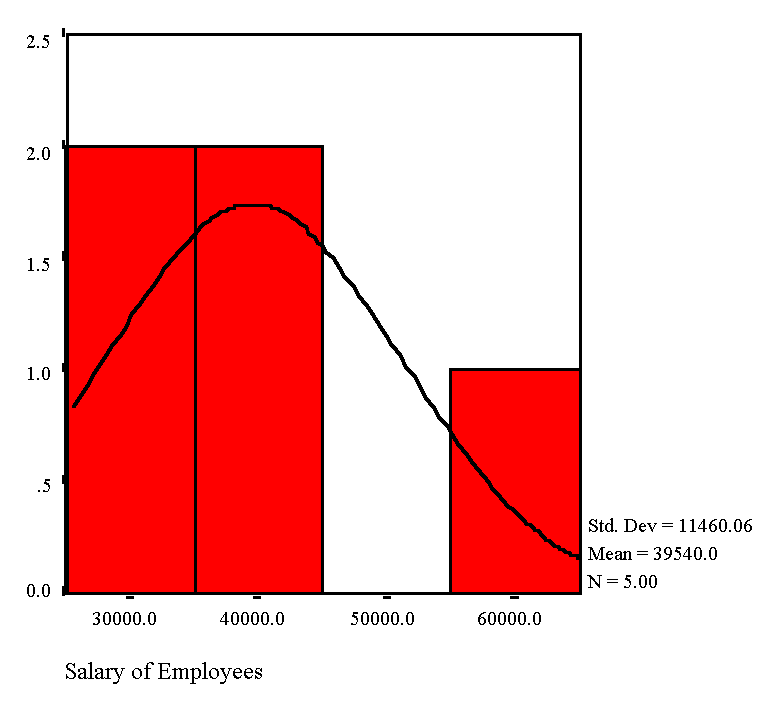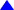### A Histogram of Employees' Salary - without a Normal CurveThis output is based on the data set of Exercise 1 (Table 2) in the SPSS/win Primer. Please note the following:

1. The sample mean of employee salary is \$39,540.00

2. The sample standard deviation of employee salary is \$11,460.06

3. The values on the horizontal axis are the mid-point values for each class interval with a class size of \$10,000.

4. The values on the vertical axis denote the number of employees; the height of each bar represents the number of employees that belong in that class interval. For instance, in the first class interval, two employees earn between \$25,000 and \$35,000; the mid-point salary is \$30,000. These individuals are, respectively, the female employee with a BA degree and 4 years of experience, and the male employee with MBA and two years of experience.

5. No employee earns a salary between \$45,000 and \$55,000.

6. The bars representing each class interval are connected because SALARY is a continuous variable.

7. The sample size n = 5; SPSS/win program uses N as the default notation for the number of observ ations. This may be confusing since N is the standard notation for the population size.

8. The histogram with a normal curve syggests the presence of positive skewness in the data.

A Histogram of Employees' Salary - with a Normal curveTop or Back to Descriptive Statistics or Exercises in the SPSS/win Primer or Home Page or Send me your Comments via E-mail